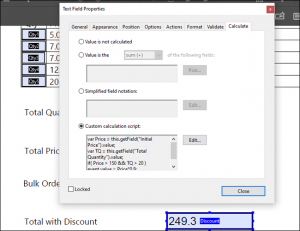## How to Create Conditional Formulas in Adobe Acrobat

Configurare noua (How To)

### Situatie

There are several ways to create complex calculations in a clean elegant PDF file. Here’s how to integrate conditional formulas into your next document.

## Conditional Formulas in Acrobat

We’ve previously covered the basics of automatic calculations in Adobe Acrobat. Conditional formulas let you go beyond the basics, creating more complex formulas in a PDF.

If you use formulas in Excel or Google Sheets, you likely already know what conditional formulas are. Also called logical formulas, they display a particular value or activate a calculation if a certain condition is met, such as if a number is negative or if a score is above the passing grade. Some of the most common conditional formulas in excel include the standard “IF,” as well as “SUMIF,” and COUNTIF.” There is also conditional formatting, where changes to the format are made if a cell meets a certain condition.

Using calculated fields, these kinds of formulas can also be applied in Adobe PDFs. You can display a number, text, or run a calculation based on the results of another input box. You can also use them in conjunction with other calculated fields. Conditional formulas are useful for many types of PDF forms, such as:

• Financial Documents: For example, if the principal determines someone’s interest rate, a conditional formula can be used to display the corresponding price based on the amount they input.
• Tests and Exams: You can make an assessment that automatically shows either “pass” or “fail” at the end based on the test taker’s total score.
• Sales: If you’re using this for transactions, you can make a box that recommends products depending on a prospective buyer’s answers to specific questions.

Take note that while calculated fields can only be made in Adobe Acrobat, the actual calculations will display in any PDF reader.

## Creating Simple Conditional Statements

Adobe Acrobat’s custom calculated fields use javascript as a programming language. Fortunately, you don’t need to know how to program in javascript to create a simple conditional field; you just need a basic pattern to follow.

In the following example, we’ll be using this simple company order form. This currently has seven fields: one for quantities of each of the five products, the total quantity, and the total price.A conditional statement in Adobe javascript follows this basic syntax:

```var variable name = this.getField("name of field").value; if( variable name condition) event.value = true result else event.value = false result```

The first line defines the value that will be used for your conditional formula. In this particular case, we used the “this.getField” to obtain the value of one of the other fields in the document. On the second line, we define the condition. Similar to excel, we specify the condition as being greater than, less than, or equal to a particular value.

Lastly, we define the results. The true result is the value that will be displayed if the condition is met. On the next line, we use “else” to generate the false result, which is the value that will be displayed if the condition is not met.

To put this into practice, we’ve created a calculated field called “Bulk Order.”  To enter a custom calculated script, right-click the chosen field in Acrobat, and select “Properties.” From here, go to “Calculate > Custom Calculation Script > Edit.”This field will determine whether or not an order counts as bulk. The box will display “Yes” if the total quantity is greater than 20 items, and “No” if it is less than 20. Considering these conditions, we have the formula:

```var TQ = this.getField("Total Quantity").value; if( TQ > 20) event.value = "Yes" else event.value = "No"```

In the image above, you can see that we set the variable name to “TQ,” and pulled the value of TQ from the field “Total Quantity.” Take note that these fields are case-sensitive. Then, we set our condition, which is that TQ must be greater than 20. If it meets this condition, it will display “Yes.” Otherwise, the box will generate “No.”If we ordered a total of 11 Lightning Cables and 10 Battery Banks, for example, we’d have a total of 21 items. It would then be considered a bulk order, and generate the following result:## Multiple Conditions

There are cases where you may want to have multiple conditions met instead of just one. Fortunately, there is a way to create a conditional field that generates values based on multiple conditions.Let’s say that in your store, all orders that reach at least 20 products and have a total price of 150 are eligible for a discount of 10%. The final amount would appear in a field called “Total With Discount.” In that case, we’d have to specify two variables and two conditions. We would have the following field:

```var Price = this.getField("Initial Price").value; var TQ = this.getField("Total Quantity").value; if( Price > 150 && TQ > 20 ) event.value = Price*0.9; else event.value = Price;```

As you can see, we defined two variables on two separate lines. You will also have to use the “&&” notation to combine the two different conditions. Take note that the final value is also a calculation that takes into account the discount.

Therefore, if we use the same total as the example above, we’d generate the following result:## Calculation Orders

One important consideration you should make is the calculation order. Unlike Excel, which renders calculations simultaneously, Acrobat relies on the user to determine which formulas come first.

To set the calculation order, go to the “Edit Form” sidebar and navigate to More > Set Field Calculation Order. In the above example, because the formulas for Bulk Order and Total With Discount are both reliant on Total Quantity and Total Price, we want to make sure that TQ and TP are calculated first.Make sure to review the calculation order before publishing your form. It’s a good idea to try a few sample inputs on your form to make sure everything is working correctly.

Permanent

### Voteaza

(9 din 19 persoane apreciaza acest articol)